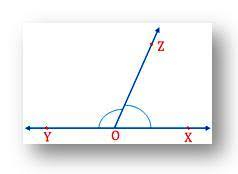# What are adjacent angles and linear pair angles and what is the difference between them?

Difference between adjacent angles and linear pair angles:

 Adjacent Angles Linear pair angles Two angles are adjacent when they have a common side and a common vertex (corner point). Example:Here angles A and B are adjacent.The angles are said to be linear if they are adjacent to each other after the intersection of the two lines.The sum of angles of a linear pair is always equal to 180°. Such angles are also known as supplementary angles. One angle is called the supplement of the other.Example: